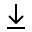MathBin Polygon Member

A polygon is a two-dimensional object that exists in two dimensions or higher. A polygon is bounded by straight line segments that form a closed loop. The segments are called edges or sides, and the points where two edges meet are called vertices or corners. The interior of a polygon is sometimes called its body.

A regular polygon has equal angles and equal sides.

Bins in Two Dimensions